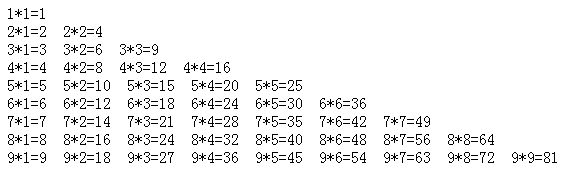# JavaScript编写九九乘法表（两种任选）

更新时间：2017年02月04日 17:19:18   作者：周全535201285我要评论

```<script language=javascript>
for(i=1;i<=9;i++){
for(j=1;j<=9;j++){
document.write (i+"*"+j+"="+i*j+" ");
if(i==j)
{document.write ("<br/>"); break;} //用的if语句，如果这两个数字相同了 那么跳出，另起一行
}
}
</script>```

```<script language="javascript">
var str=" "
for(var i=1;i<=9;i++)
{
for(var j=1;j<=i;j++)
{
str+=i+"*"+j+"="+i*j+" ";
//拼接字符串
}
str+="<br />"
}
document.write(str);
</script>
```